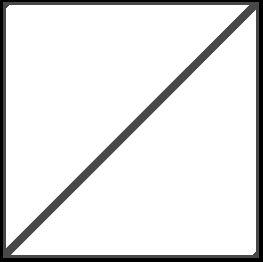100 posts
OpenGL: still a a bit confused with meshes/models
Edited by da447m on

Let me call here a "mesh" a set of points, with a set of texture coordinates and indexes.

Let's say points is "where", texcoords is "what" and indexes is "how" (and the "how" is always telling how to draw triangles as I understand).

This will be paired with the function

glDrawElements(GL_TRIANGLES, idx.count, GL_UNSIGNED_BYTE, (GLvoid*)0);



Then, let's put here the most simple platonic solid, the tetrahedron

struct Plato::Tetrahedron
{
std::vector<GLfloat> points =
{
#if 1
1, 1,-1, // A
1,-1, 1, // B
-1, 1, 1, // C

1, 1,-1, // A
1,-1, 1, // B
-1,-1,-1, // D

1, 1,-1, // A
-1, 1, 1, // C
-1,-1,-1, // D

1,-1, 1, // B
-1, 1, 1, // C
-1,-1,-1  // D
#else
1, 1,-1, // A
1,-1, 1, // B
-1, 1, 1, // C
-1,-1,-1  // D
#endif

};

std::vector<GLfloat> texcoords =
{
0, 0,
1, 0,
1, 1,

0, 0,
1, 0,
1, 1,

0, 0,
1, 1,
0, 1,

0, 0,
1, 1,
0, 1
};

std::vector<GLbyte> indexes =
{
/// ACB
0,2,1,
/// ABD
3,4,5,
6,8,7,
/// BCD
9,10,11
};
};


Let me put here the texture so you can understand the coords:This is just a test of course.

So here is my first question:

1 - I'd like to use the simpler set of points, the problem is that it seems that texture coords HAVE TO be such that each x,y,z point has a corresponding s,t texture coordinate pair. Is that true? If so I cannot use the economical set of points, but must always repeat points to make triangles;

I'm following Anton's OpenGL tutorials where he says points for triangles/squares must go counter clockwise, fair enough, so notice that I also must revert the order of triangles in the indexes if they are facing the back of my initial view.

Say I have ABC triangle (which is accounted for in point in counter clockwise fashion), if this triangle is 180° rotated on the y axis, facing backwards, then I must get the points ACB; this is reflected on my indexes ACB and ADC, without this a blank is drawn.

So here's my second question:

2 - is there a way to simplify/generalize how to do these meshes? I can see some parts can be automated, but ordering by hand the order of points depending on my model is a nightmare. There must be a way of doing these things faster?!

Thanks folks.

Mārtiņš Možeiko
2540 posts / 2 projects
OpenGL: still a a bit confused with meshes/models
1. When draw call is issued GPU processes vertices independently with vertex shader. Each vertex is set of attributes - position, texcoord, normal, etc... Don't confuse "position" with "vertex". Position is your vec3 (or vec2). Vertex is multiple vecN's, each with different meaning.

I don't know what you mean by "economical set of points". The index buffer is there so you can reuse vertices for triangles. If triangles share same vertex - you use same index. Saves memory and performance.

"Counter clockwise" thing matters only if you have enabled back face culling. Clockwise direction is how GL knows whether polygon is facing you, or facing backwards and can be discarded. You can flip clockwise direction for front vs back faces with glFrontFace function.

1. The automation happens when you use 3D software - Blender, Maya, etc... They create these vertex/index buffers for you. You just "draw" the model.
100 posts
OpenGL: still a a bit confused with meshes/models

Hi Martins, thank you.

"economical set of points".

just using the bare minimum of points in the space, since I assume i can define indexes to pick any 3 of those points and form triangles

"Counter clockwise" thing matters only if you have enabled back face culling. Clockwise direction is how GL knows whether polygon is facing you, or facing backwards and can be discarded.

Oh, so there is nothing special about that? So why is Anton using counter clockwise? Is there any advantage in using back face culling?

Mārtiņš Možeiko
2540 posts / 2 projects
OpenGL: still a a bit confused with meshes/models